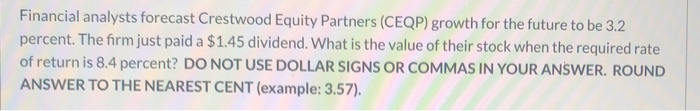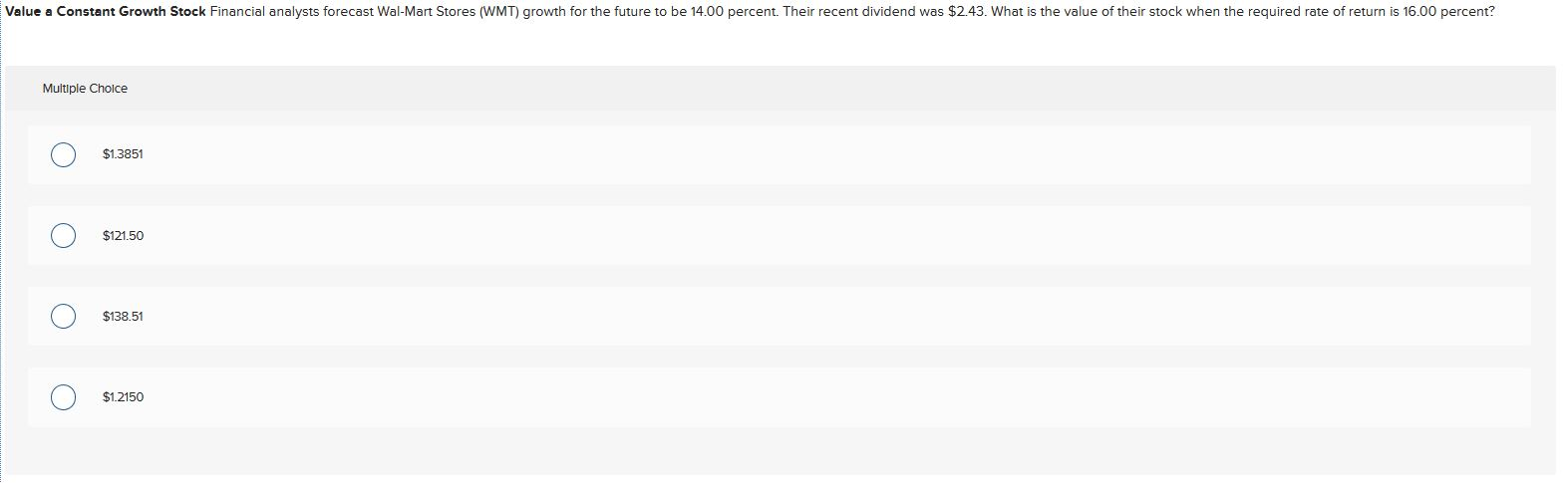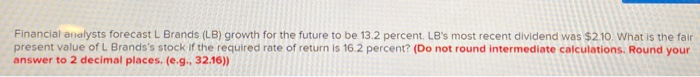Question

Financial analysts forecast Crestwood Equity Partners (CEQP) growth for the future to be 3.1 percent. The firm just paid a \$1.22 dividend. What is the value of their stock when the required rate of return is 13.9 percent?

Ans \$ 11.65

 P0 = Price of Share D1 = Current Dividend Ke = Cost of Equity g = growth rate P0 = D1 / (Ke - g) P0 = 1.2578 / (13.9%- 3.1%) P0 = 11.65 D1 = D0* (1 + g) D1 = 1.22* (1 + 3.1%) D1 = 1.2578

#### Earn Coins

Coins can be redeemed for fabulous gifts.

Similar Homework Help Questions
• ### Financial analysts forecast Crestwood Equity Partners (CEQP) growth for the future to be 3.2 percent. The...Financial analysts forecast Crestwood Equity Partners (CEQP) growth for the future to be 3.2 percent. The firm just paid a \$1.45 dividend. What is the value of their stock when the required rate of return is 8.4 percent? DO NOT USE DOLLAR SIGNS OR COMMAS IN YOUR ANSWER. ROUND ANSWER TO THE NEAREST CENT (example: 3.57).

• ### Value a Constant Growth Stock Financial analysts forecast Wal-Mart Stores (WMT) growth for the future to...

Value a Constant Growth Stock Financial analysts forecast Wal-Mart Stores (WMT) growth for the future to be 11.00 percent. Their recent dividend was \$1.53. What is the value of their stock when the required rate of return is 16.00 percent?

• ### Value a Constant Growth Stock Financial analysts forecast Best Buy Company (BBY) growth for the future...

Value a Constant Growth Stock Financial analysts forecast Best Buy Company (BBY) growth for the future to be 16.00 percent. Their recent dividend was \$1.79. What is the value of their stock when the required rate of return is 17.23 percent?

• ### Value a Constant Growth Stock Financial analysts forecast Wal-Mart Stores (WMT) growth for the future to...Value a Constant Growth Stock Financial analysts forecast Wal-Mart Stores (WMT) growth for the future to be 14.00 percent. Their recent dividend was \$2.43. What is the value of their stock when the required rate of return is 16.00 percent? \$1.3851 \$121.50 \$138.51 \$1.2150

• ### Value a Constant Growth Stock Financial analysts forecast Wal-Mart Stores (WMT) growth for the future to...

Value a Constant Growth Stock Financial analysts forecast Wal-Mart Stores (WMT) growth for the future to be 12.00 percent. Their recent dividend was \$1.83. What is the value of their stock when the required rate of return is 16.00 percent? Multiple Choice \$45.75 \$.5124 \$.4575 \$51.24

• ### Financial analysts forecast Safeco Corp.’s (SAF) growth rate for the future to be 7 percent. Safeco’s recent dividend wa...

Financial analysts forecast Safeco Corp.’s (SAF) growth rate for the future to be 7 percent. Safeco’s recent dividend was \$1.80. What is the value of Safeco stock when the required return is 9 percent? (Round your answer to 2 decimal places.)

• ### Financial analysts forecast L Brands (LB) growth for the future to be 13.2 percent. LB's most...Financial analysts forecast L Brands (LB) growth for the future to be 13.2 percent. LB's most recent dividend was \$2.10. What is the fair present value of L Brands's stock if the required rate of return is 16.2 percent? (Do not round intermediate calculations. Round your answer to 2 decimal places. (e.g. 32.16))

• ### Financial analysts forecast startup company ABC Inc.’s growth for the future will be 12%. But, they...

Financial analysts forecast startup company ABC Inc.’s growth for the future will be 12%. But, they believe that ABC won’t pay its first dividend until three years from now. They expect that first dividend will be \$0.30. What is the value of ABC stock today when the required return is 15%?

• ### financial analyst forecast Safeco core growth rate for the future to be 12% Safeco is recent...

financial analyst forecast Safeco core growth rate for the future to be 12% Safeco is recent dividend was 1.65 what is the value of Safeco stock when the required return is 14%

• ### 3. Problem 8-20 Value a Constant Growth Stock (LG8-5) Financial analysts forecast Limited Brands (LTD) growth...

3. Problem 8-20 Value a Constant Growth Stock (LG8-5) Financial analysts forecast Limited Brands (LTD) growth rate for the future to be 11.5 percent. LTD’s recent dividend was \$0.60. What is the value of Limited Brands stock when the required return is 13.5 percent? (Round your answer to 2 decimal places.) 8. Problem 8-32 Changes in Growth and Stock Valuation (LG8-5) Consider a firm that had been priced using an 8.5 percent growth rate and a 10.5 percent required return....